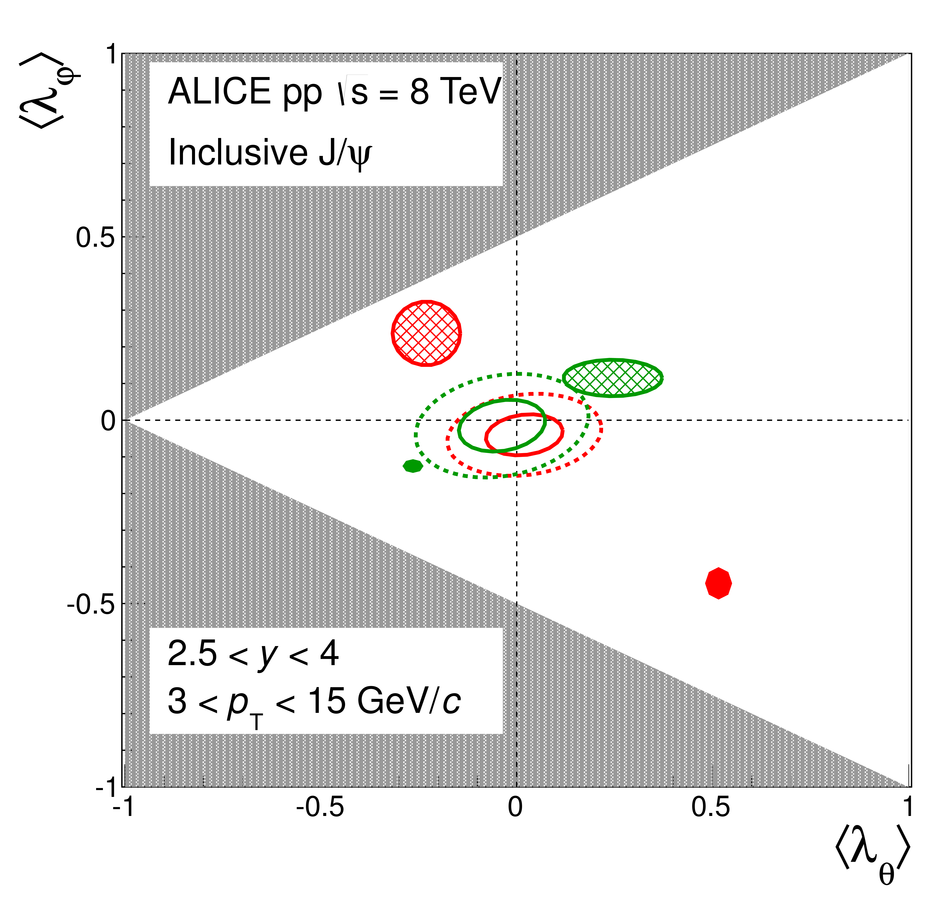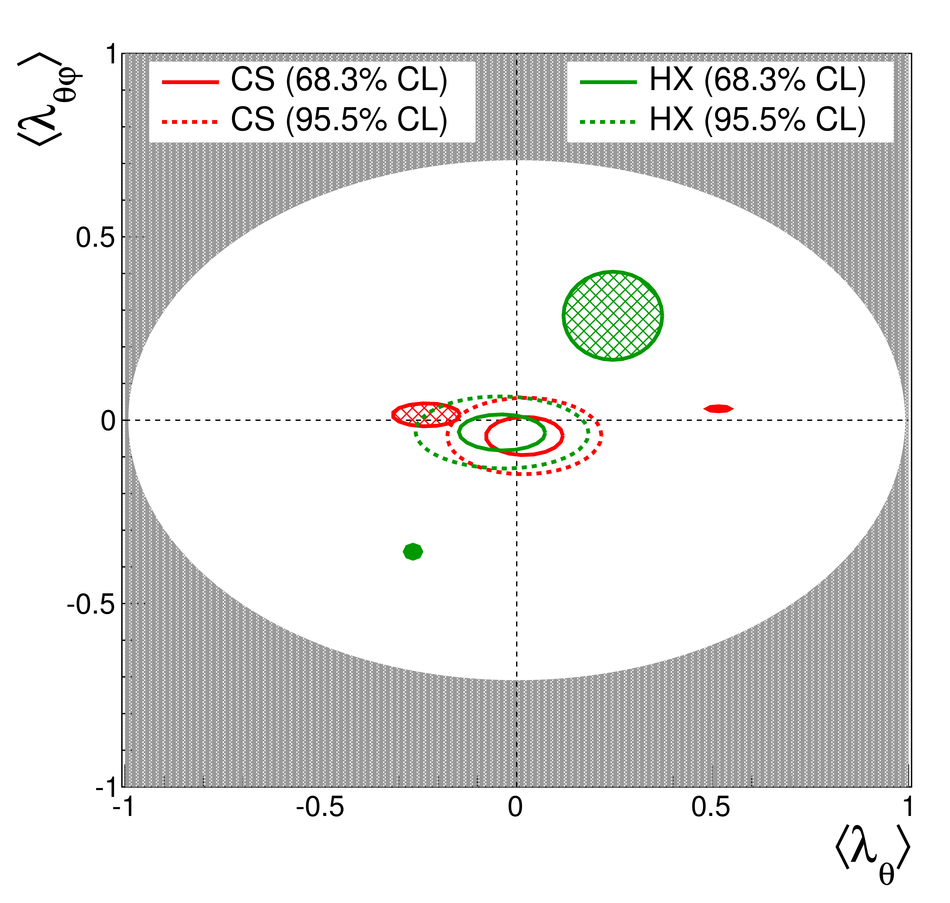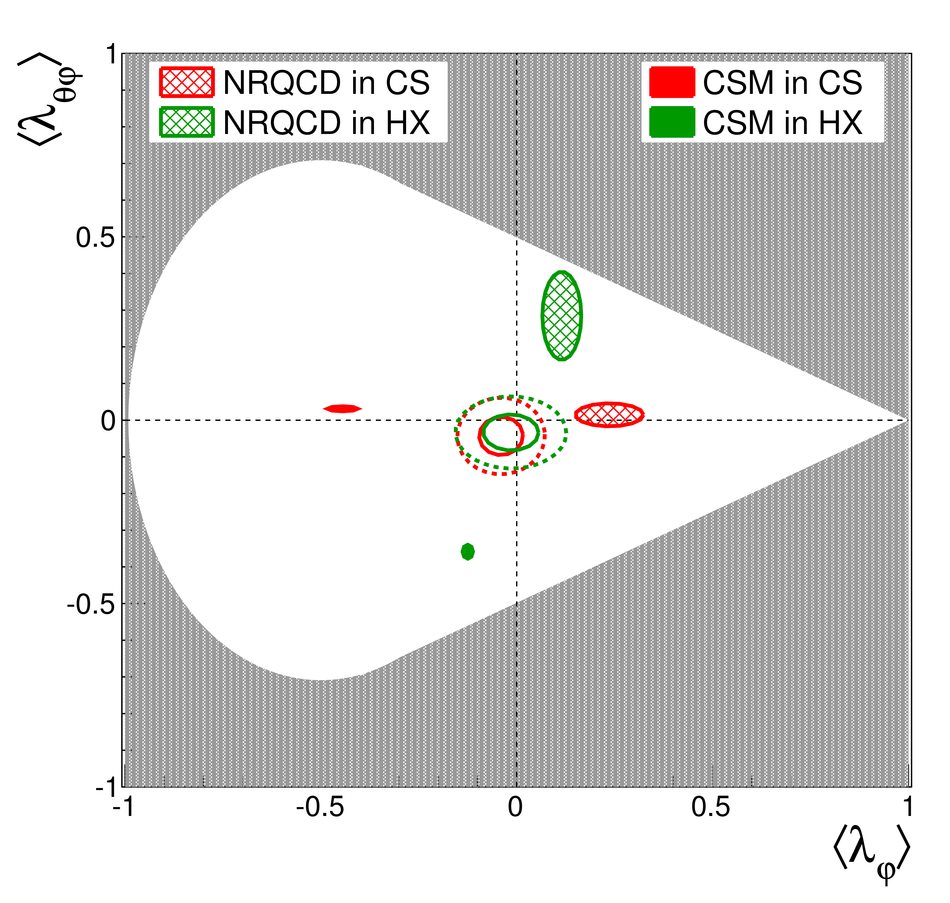Measurement of the inclusive J/$ψ$ polarization at forward rapidity in pp collisions at $\sqrt{s} = 8$ TeV

We report on the measurement of the inclusive J/$\psi$ polarization parameters in pp collisions at a center of mass energy $\sqrt{s} = 8$ TeV with the ALICE detector at the LHC. The analysis is based on a data sample corresponding to an integrated luminosity of 1.23 pb$^{-1}$. J/$\psi$ resonances are reconstructed in their di-muon decay channel in the rapidity interval $2.5 <~ y <~ 4.0$ and over the transverse-momentum interval $2 <~ p_{\rm T} <~ 15$ GeV/$c$. The three polarization parameters ($\lambda_\theta$, $\lambda_\varphi$, $\lambda_{\theta\varphi}$) are measured as a function of $p_{\rm T}$ both in the helicity and Collins-Soper reference frames. The measured J/$\psi$ polarization parameters are found to be compatible with zero within uncertainties, contrary to expectations from all available predictions. The results are compared with the measurement in pp collisions at $\sqrt{s} = 7$ TeV.

Figures

Figure 1

 ($A$$\times$$\epsilon$) 2-D maps in the planes ($\cos\theta$, $p_{\rm T}$) (left) and ($\varphi$, $p_{\rm T}$) (right) in the Collins-Soper frame. The plots illustrate the symmetry with respect to $\cos\theta = 0$ (left) and with respect to $\varphi = \pi$ and $\pi/2$ (right), while the grid shows the binning used to build the $W_1(\cos\theta)$ and $W_2(\varphi)$ distributions in each $p_{\rm T}$ range.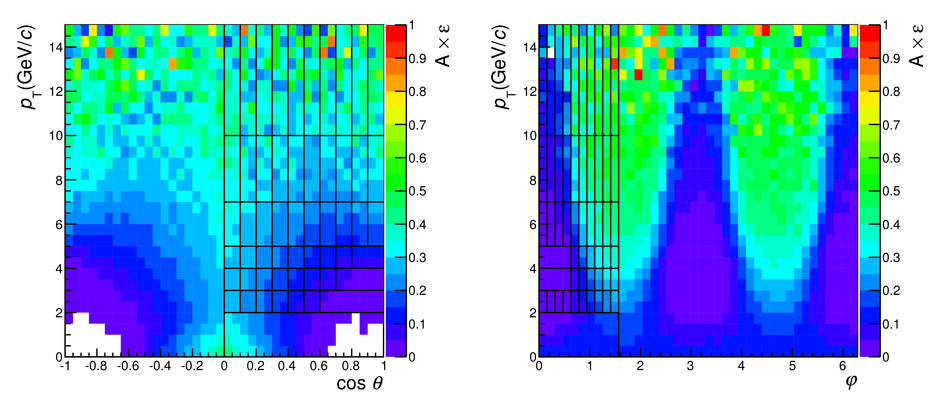Figure 2

 Acceptance corrected angular distributions of J/$\psi$ reconstructed in the di-muon decay channel $W_1(\cos\theta)$, $W_2(\varphi)$ and $W_3(\widetilde{\varphi})$ in the helicity frame for the transverse momentum interval $4 < p_{\rm T} < 5$~\GeVc, together with the results of the simultaneous fit (see text for details). Vertical bars correspond to statistical uncertainties.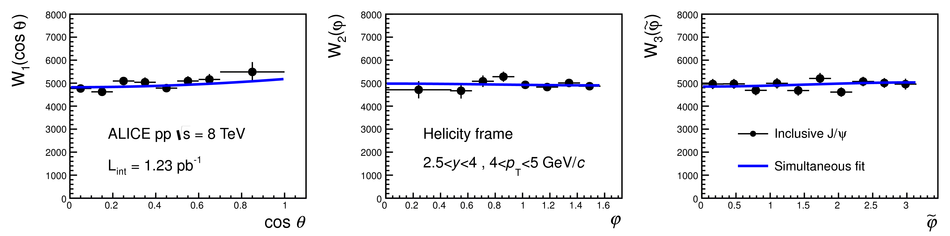Figure 3

 ALICE inclusive J/$\psi$ polarization parameters in pp collisions at $\sqrt{s} = 8$~TeV (black points) compared with ALICE~ inclusive J/$\psi$ (orange squares, shifted horizontally by $- 0.3$~\GeVc) and LHCb~ prompt J/$\psi$ (blue open diamonds, shifted horizontally by $+ 0.3$~\GeVc) measurements at $\sqrt{s} = 7$~TeV in the rapidity interval $2.5 < y < 4.0$. The error bars represent the total uncertainties. Left and right plots show results in the Collins-Soper and helicity frames, respectively, for $\lambda_\theta$ (top plots), $\lambda_\varphi$ (middle plots) and $\lambda_{\theta\varphi}$ (bottom plots).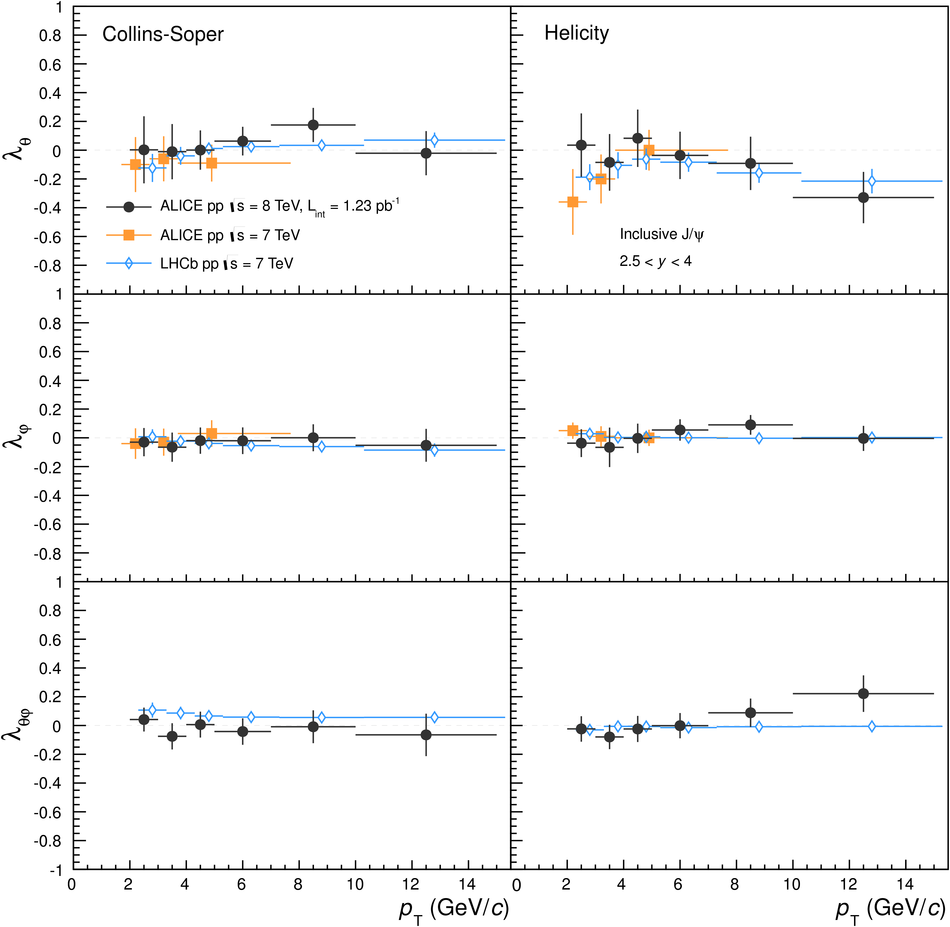Figure 4

 Inclusive J/$\psi$ polarization parameters in pp collisions at $\sqrt{s} = 8$~TeV (black points, error bars represent the total uncertainties) compared with model predictions: NLO CSM~ (blue filled bands), NRQCD~ (red shaded bands) and NRQCD2~ (light blue hatched band). Left and right plots show the results in the Collins-Soper and helicity frames, respectively, for $\lambda_\theta$ (top plots), $\lambda_\varphi$ (middle plots) and $\lambda_{\theta\varphi}$ (bottom plots).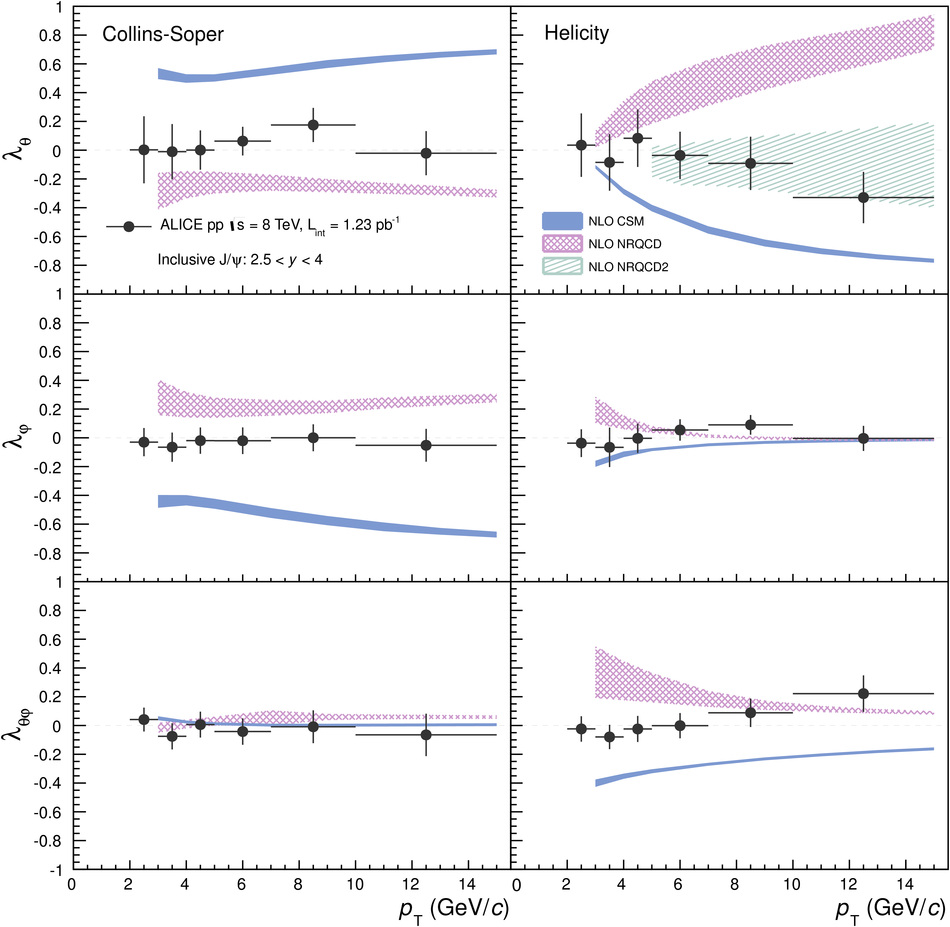Figure 5

 Inclusive J/$\psi$ frame-invariant quantity $\widetilde{\lambda}$ in pp collisions at $\sqrt{s} = 8$~TeV in the Collins-Soper (red points, shifted horizontally by $-0.1$~\GeVc) and helicity (green squares, shifted horizontally by $+0.1$~\GeVc) frames compared with the NLO CSM (blue full band) and NRQCD (red shaded band) model predictions from~.Figure 6

 Average $p_{\rm T}$-integrated (in rapidity range $2.5 < y < 4.0$) inclusive J/$\psi$ polarization parameters $\langle\lambda_\theta\rangle$, $\langle\lambda_\varphi\rangle$ and $\langle\lambda_{\theta\varphi}\rangle$ in allowed 2-D regions (white areas) for $3 < p_{\rm T} < 15$~\GeVc. Full (dashed) ellipses show 1-$\sigma$ (2-$\sigma$) contours in Collins-Sopper (CS, red) and helicity (HX, green) frames. Model predictions~ are represented by filled contours, full filled for the CSM and shaded filled for the NRQCD model, in green for the HX frame and in red for the CS frame.